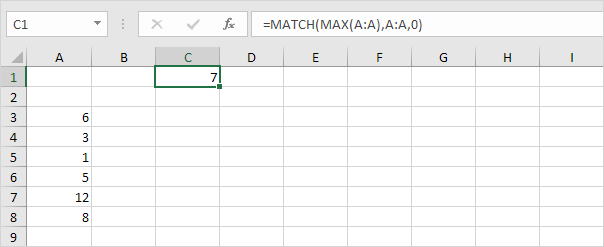# Locate Maximum Value

This example teaches you how to find the cell address of the maximum value in a column.

1. First, we use the MAX function to find the maximum value in column A.2. Second, we use the MATCH function to find the row number of the maximum value.Explanation: the MATCH function reduces to =MATCH(12,A:A,0), 7. The MATCH function returns the position of the maximum value in column A. Set the third argument to 0 to return an exact match.

3. Finally, we use the ADDRESS function to return the cell address.Explanation: the ADDRESS function reduces to =ADDRESS(7,1), \$A\$7. The first argument specifies the row number. The second argument specifies the column number.

Previous articleHow to use GETPIVOTDATA in Excel
Next articleHow to Lock Cells in Excel